Courses

# Moment of a Force (Scalar Formulation) Mechanical Engineering Notes | EduRev

## Mechanical Engineering : Moment of a Force (Scalar Formulation) Mechanical Engineering Notes | EduRev

The document Moment of a Force (Scalar Formulation) Mechanical Engineering Notes | EduRev is a part of the Mechanical Engineering Course Engineering Mechanics - Notes, Videos, MCQs & PPTs.
All you need of Mechanical Engineering at this link: Mechanical Engineering

Moment of a force: Scalar Formulation

The magnitude of the moment of a force:

MO: Magnitude of the moment of F around point O

d: Perpendicular distance from O to the line of action of F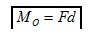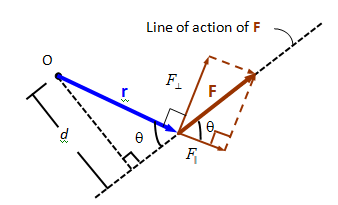Note: moving a force along its line of action does not change its moment

Note: M0 =Fd =Fr sin (θ) =rF⊥

Direction of the moment in 2-D:The direction of the moment is given by the right hand rule: Counter Clockwise (CCW) is out of the page, Clockwise (CW) is into the page.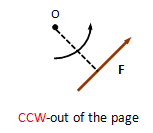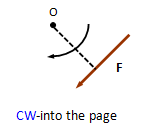Calculating the moment in 2-D using components: Moments add together as vectors. Select a positive direction (CCW or CW), then calculate each moment and add them using the proper sign for each term. For example:

M0 =-Fx d1 + Fy d2 CCW positive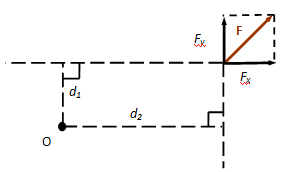Offer running on EduRev: Apply code STAYHOME200 to get INR 200 off on our premium plan EduRev Infinity!

,

,

,

,

,

,

,

,

,

,

,

,

,

,

,

,

,

,

,

,

,

;